Courses

# Test: Environmental Engineering- 3

## 10 Questions MCQ Test GATE Civil Engineering (CE) 2022 Mock Test Series | Test: Environmental Engineering- 3

Description
This mock test of Test: Environmental Engineering- 3 for Civil Engineering (CE) helps you for every Civil Engineering (CE) entrance exam. This contains 10 Multiple Choice Questions for Civil Engineering (CE) Test: Environmental Engineering- 3 (mcq) to study with solutions a complete question bank. The solved questions answers in this Test: Environmental Engineering- 3 quiz give you a good mix of easy questions and tough questions. Civil Engineering (CE) students definitely take this Test: Environmental Engineering- 3 exercise for a better result in the exam. You can find other Test: Environmental Engineering- 3 extra questions, long questions & short questions for Civil Engineering (CE) on EduRev as well by searching above.
QUESTION: 1

### Which sewer is having the maximum fluctuations in sewer discharge?

Solution:

The fluctuation in sewage discharge is found to be maximum in the lateral sewer.

The fluctuation in sewage discharge reduced from lateral sewer to branch sewer, from branch sewer to the main sewer and from the main sewer to outfall sewer. An example of the respective fluctuation values are given below: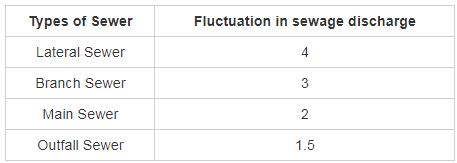QUESTION: 2

### The Maximum velocity through a circular channel takes place when the depth of flow is equal to:

Solution:

Maximum velocity occurs at 0.81 times the diameter and maximum discharge occurs at 0.95 times the diameter.

QUESTION: 3

### For the Sewer of size 550 mm, it is designed to run “x” times full. The value of x is

Solution:

Sewers are never designed to run at full depth. If the size of the Sewer is less than 400 mm, these are designed to run half full. If the Sewer size is in the range of 400 to 900 mm, these are designed to run 2/3rd full. When the size of Sewer is more than 900 mm, these are designed for 3/4th full.

QUESTION: 4

Consider the following statements for Sewers

1. Maximum Daily Discharge is two times average daily discharge

2. Maximum Hourly Discharge is three times average daily discharge

3. Minimum Daily discharge is Half of the average daily discharge

4. Minimum Hourly discharge is 2/3rdof the average daily discharge.

Identify the incorrect Statement?

Solution:

(i) Maximum Daily discharge = 2 × Average Daily Discharge

(ii) Maximum Hourly discharge = 3 × Average Daily Discharge

(iii) Minimum Daily discharge = 2/3 × Average Daily Discharge

(iv) Minimum Hourly discharge = 1/3× Average Daily Discharge

QUESTION: 5

Two pipe system of providing building drainage consists of

Solution:

In this system, two sets of vertical pipes are laid, i.e., one for draining night soil, and the other for draining sullage. The pipes of the first set carrying night soil are called soil pipes, and the pipes of the second set carrying sullage from baths etc., are called sullage pipes or waste pipes. The soil pipe as well as the waste pipe, are separately ventilated by providing separate vent pipes or antisiphonage pipes.

QUESTION: 6

The slope of a 1.0 m diameter concrete sewer laid at a slope of 1 in 1000, develops a velocity of 1 m/s, when flowing full. When it is flowing half – full, the velocity of flow through the sewer will be

Solution:

For,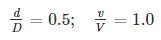Where d is depth of flow

v is flow velocity at depth d

D is diameter of sewer

V is flow velocity at full flow

∴ v = 1.0 m/s

QUESTION: 7

A watershed of area 90 hectare has a runoff coefficient of 0.4. A storm of duration larger than the time of concentration of the watershed and of intensity 4.5 cm/h creates a peak discharge of

Solution:

Peak flood by rational formula is given by QP = 136 KPcA
Where, K = Co-efficient of run off; pc = intensity (cm/hr); A = area in hectare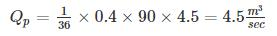QUESTION: 8

The drainage discharge of a town of 10 hectares area, consisting of 50% cc paved (k = 1.0), 30% unpaved (k = 0.20) and remaining as wooded (k = 0.10) with a maximum rain intensity of 5 cm/hr, would be computed by Rational formula, as equal to ----

Solution:

Overall, co-efficient of runoff (K or I)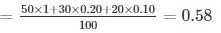Discharge, Q = AIR/360

A = Area in hectares

R = Intensity of rainfall in mm/hr

I = Co-efficient of run-off.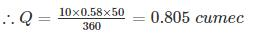*Answer can only contain numeric values
QUESTION: 9

In a city of population 2 Lakhs, the average per capita water demand is 135 L/c/day. To provide with the sewerage facilities in the town sewer is to be designed. Calculate the design discharge (m3/s) through the branch sewer if it is given that sewer is designed to carry 3 times the average discharge. Assume 80% of the water supplied in the city enters in the sewer

Solution:

About 80% of the water supplied in the city enters in the Sewer.

Average discharge through the Sewer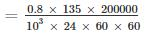QAvg = 0.25 m3/sec

QDesign = 3 × QAvg

QDesign = 3 × 0.25 = 0.75 m3/sec

QDesign = 0.75 m3/sec

*Answer can only contain numeric values
QUESTION: 10

Calculate the minimum velocity (m/sec) required to transport Coarse sand through a sewer of 35 cm diameter with sand particles of 0.97 mm diameter and specific gravity 2.59, and organic matter of 4.75 mm average size with a specific gravity of 1.42. The friction factor for the sewer material may be assumed as 0.04 and roughness coefficient of 0.013. Consider k = 0.05 for inorganic solids and 0.07 for organic solids.

Solution: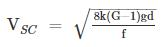Where Vsc = self cleaning velocity or minimum velocity in m/sec

k = constant depend upon type of Particle present in the Sewage

f = friction factor

d = size of particle under consideration(m)

For inorganic solid i.e. Coarse sand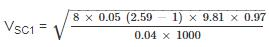VSC1 = 0.389 m/sec

For organic solidVSC2 = 0.523 m/s

Minimum velocity required will be maximum of VSC1 and VSC2

So, VSC = 0.523 m/sec